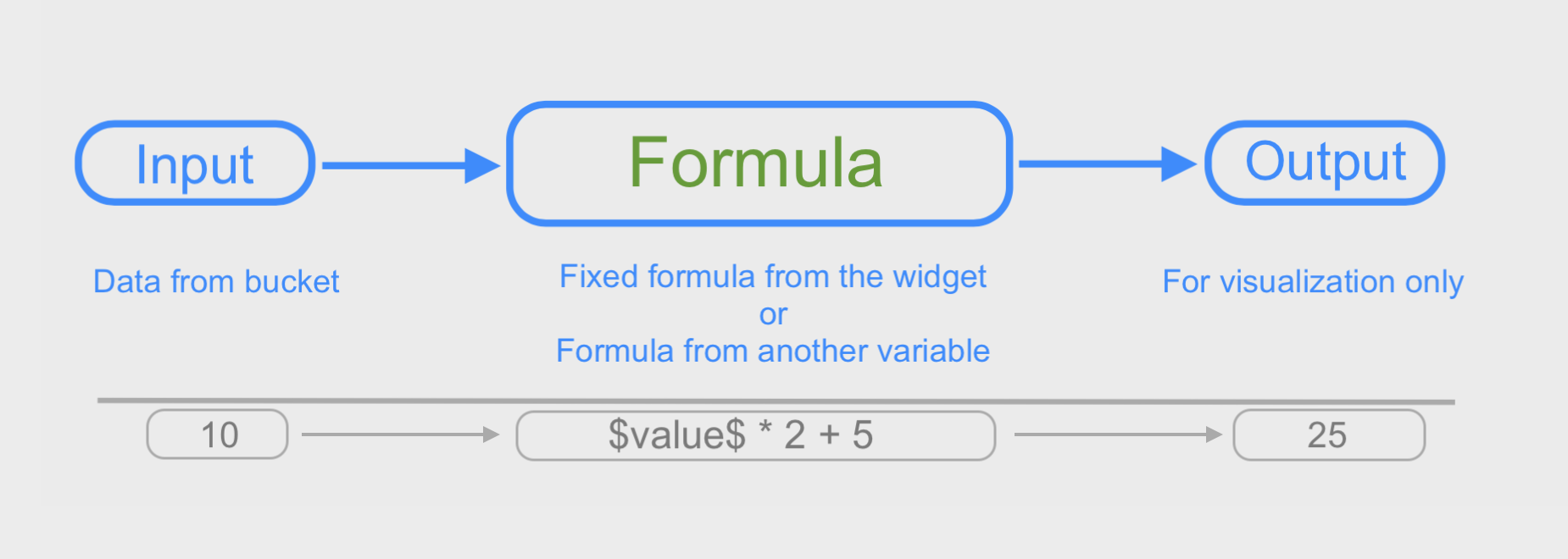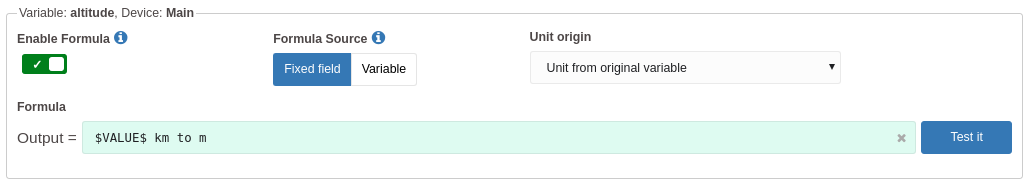You can use Formula to modify the visualization of data in certain widgets. Formula is located inside the configuration tab of most widgets, and it can be applied to all fields of a stored register, including: variable, value, unit, serie, location, and metadata.• The input is the data from the bucket that you defined as the variable.
• The formula will be applied to modify the input. It can be 'fixed' (edited in the Formula tab), or it can come from another 'variable' so that it can be changed dynamically if necessary.
• The output is the temporary value that will be displayed in the widget.

Notice that Formula doesn't modify any data in your buckets, it only modifies the visualization.

You can get a preview of the output of your formula by clicking on the 'Test it' button.### Source of the formula

You have the option to enter the formula into the fixed field, or you may select a variable that will contain the formula in the JSON field 'value'. If you select the fixed field, you will enter the formula directly in the widget.

If the source of your formula is coming from a variable, you will need to select that variable, and make sure that it contains the formula in its 'value' field. Also, you can add a 'unit' in that variable to use it to display later. For example, a variable that converts temperature from °F to °C would be like this:

```{
"variable": "equation_f_c",
"unit"    : "°C",
"value"   : "(\$value\$ −32)×5/9"
}```

### Operators

These are some of the operators available for you to use:

 Operators Name Example +, - add, subtract \$value\$ + 1000 /, * divide, multiply \$value\$ / 2.14 to, in unit conversion \$value\$ inch to cm ? : conditional expression \$value\$==1 ? \$VALUE\$ ON : OFF >>, << arithmetic shifts \$VALUE\$ >> 1 sqrt, sin, log math operations sqrt (\$value\$)

More options for Operators can be found here

Because not all options are available for the Formula, make sure you test it before deploying your solution.

You can also use input fields other than \$value\$; for example:

 JSON Field Example \$variable\$ '\$variable\$' \$unit\$ equalText('\$UNIT\$', 'C') ? \$VALUE\$  degC to degF : \$VALUE\$ \$serie\$ \$serie\$ \$location.lat\$ \$location.lat\$ \$location.lng\$ concat('\$location.lat\$',';','\$location.lng\$') \$metadata.\$ '\$metadata.color\$' all JSON fields \$*\$

### Formula application

Let's say that you want to visualize a 'temperature' in Fahrenheit, but your device stored it in Celsius, you can use one of these formulas:

``` \$VALUE\$ celsius in fahrenheit
```
` \$VALUE\$ degC to degF  `
` (\$VALUE\$ × 9/5) + 32`

More examples are available in the dropdown list in the Formula field.

You can see more options by accessing the Evaluate session of the math.js documentation that we used as reference.

#### Units for the output variables

In the example above, you will want to see the output value showing the unit °F, instead of the original °C because you converted the value to a new scale.

You have options to define the origin of the unit to be displayed using the field Unit Origin. Learn the options to display units here.

#### Minimum and Maximum limits

The formula will not adjust the limits of the widgets. The Min and Max values of the range are still solely controlled by the settings defined in the 'Data Range' tab.